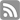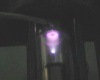# Analytical Instrument Documents

### JEOL ResourcesDocumentation in support of your JEOL product.

Non Uniform Sampling in Routine 2D Correlation Experiments

### Introduction

Data obtained from two-dimensional NMR experiments is incredibly useful for structure elucidation of complex molecules, especially when their one-dimensional spectra feature overlapping peaks. However, some experiments require significant amounts of time in order to yield data with adequate resolution or signal to noise for unambiguous interpretation. Any means of reducing the total acquisition time is useful. In this Note, we’ll explore a technique known as Non Uniform Sampling (NUS), demonstrate how it can be used to speed up data collection, and highlight how it can be employed in Delta™ on JEOL Spectrometers.

### Sampling of Indirect Dimensions

In typical 2D correlation experiments the indirect dimension is sampled as a series of 1D measurements where a delay (sometimes called the evolution time) is incremented so that the effects of a desired interaction, such as J-coupling between protons in a COSY or the proton and carbon chemical shifts in an HSQC, can be observed. The example below (Figure 1) is from an HSQC data set sampled with 128 points. The size of the steps is the inverse of the spectral width. In this case, a time step of 0.05 ms corresponds to a sweep width of 20,000 Hz, or approximately 200 ppm at 100 MHz (the frequency of 13C on a 400 MHz system). Similarly, the resolution is determined by the total time elapsed. In this particular case, 6.4 ms of total acquisition time (i.e., the longest time interval) corresponds to a resolution of 157 Hz in the indirect dimension.

As an alternative, we can collect a smaller sample of the 1D spectra in the indirect dimension in order to speed up the experiment. This technique is known as Non Uniform Sampling (NUS). In this scheme, we collect only a portion of the points (in Figure 2 below, 25%) and fill in the missing 1D increments with zeroes.

Attached Files
Showing 0 Comment